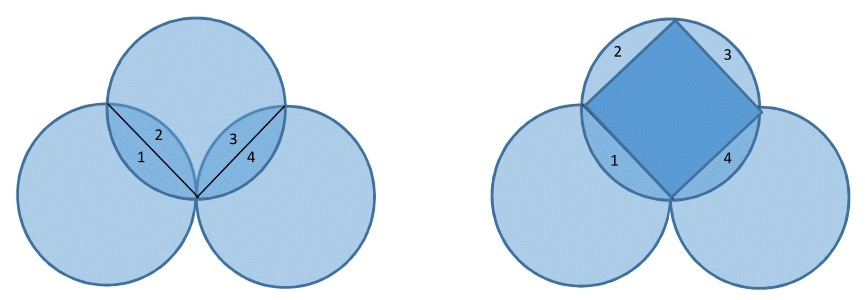# I have 3 unit circles. Two of them are externally tangent to each other. The third one passes through tangent point...

301 views

I have 3 unit circles. Two of them are externally tangent to each other. The third one passes through the tangent point, cutting two symmetrical areas from those two circles, as shown in the diagram. What is the shaded area?posted Jan 17, 2018The area of the shape is equal to the area of a square inscribed in a unit circle, that is a square with side √2 and area 2.answer Feb 3, 2018

Similar Puzzles

Three non-concentric circles of equal radii are drawn such that each circle touches the other two externally. The centres are joined to form a triangle whose area is 17320.5 cm^2. Find the area of the triangle not included in the circles.A square contains a semicircle and a quarter circle, as shown. The two circles are tangent to each other. The semicircle has a diameter equal to 12 and the quarter circle has a radius equal to 12.
What is the area of the square?
What is the length of the tangent line segment AB?In the figure if AC is a common tangent of two touching circles. If B is the touching point of both circles then which is of the following is true about it and why...

1. Angle B is a right angle
2. Angle B is an obtuse angle
3. Angle B is an acute angle
4. There is not enough information to know anything about Angle B.+1 vote

In the figure AC is a common tangent of two touching circles. If B is touching point of both circles then find angle B.